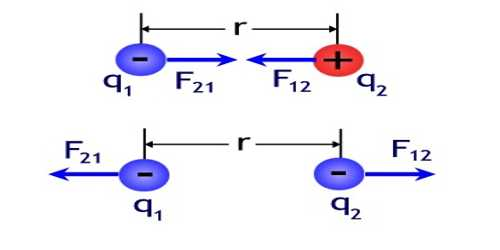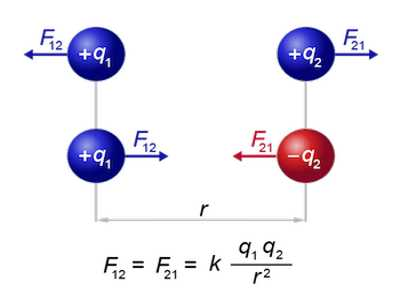Physics

# Electric ForceElectric force

In an electric field there always exists a force on a charge. If the charge is positive then the force acts along the direction of the electric field. On the other hand, if the charge is negative then the direction of the force becomes opposite.

The electric force is one of the most powerful, fundamental forces in the universe. This is the force that exists between all charged particles. The electric force is responsible for such diverse phenomena as making your hair stand up on a cold dry day, creating chemical bonds, and allowing you to see when you turn on a lamp on a dark night.If the charge is to be displaced by the force then some external cause will have to work against the force. As a result, the potential energy of the charge will increase. If a test charge + q experiences a force F at a point inside the electric field strength E. then the force acting at that point is,

F = qE

That means, the force acting on a charge placed at a point in an electric field is equal to the product of the electric field at that point and the charge placed there. A positive charge will acquire force along the direction of the field, whereas negative charge will experience the force in the opposite direction of the field. Unit of electric force is Newton.